# MATLAB applications | Mathematics Centre## MATLAB applications

Below you will find .exe apps ready to download and use. To run an .exe file you will first have to install so called Matlab Runtime, which is a set of libraries that are necessary for running apps compiled in Matalab.

Matlab Runtime version R2016b (9.1) 64-bit is available for download from the Mathworks website. Installation of Runtime is needed to be done only once.

The short tutorial below shows how to do it.

After installing Matlab Runtime one must restart their computer. Most application windows can be resized to desired dimensions.

CNMiKnO employees may also download source codes that can be run directly from Matlab command window. These are availabel on eNauczanie.

##### Limit of a sequence - definition

App shows how the definition of a limit of a sequence works.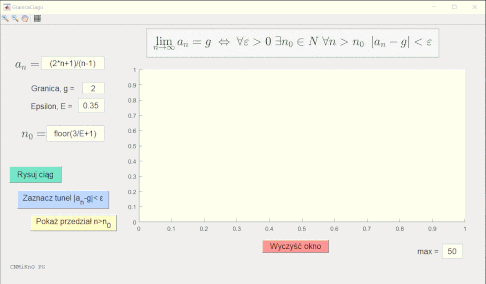##### Graphs of sine/cosine functions

App shows how sine and cosine graphs are created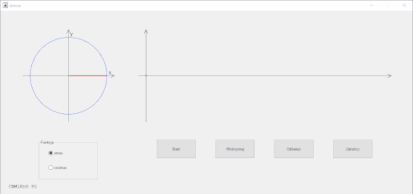##### Multiplication of Matrices

App performs multiplication of matrices up to 5x5 in size.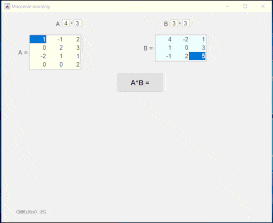##### Inverse matrix using Gaussian elimination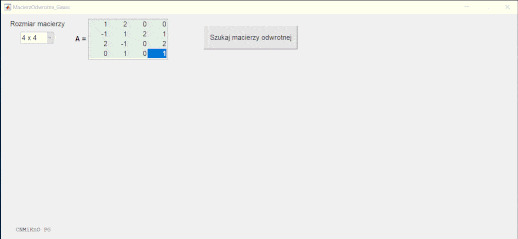##### Fourier series - graphs

App draws graphs of Fourier series for several sample functions.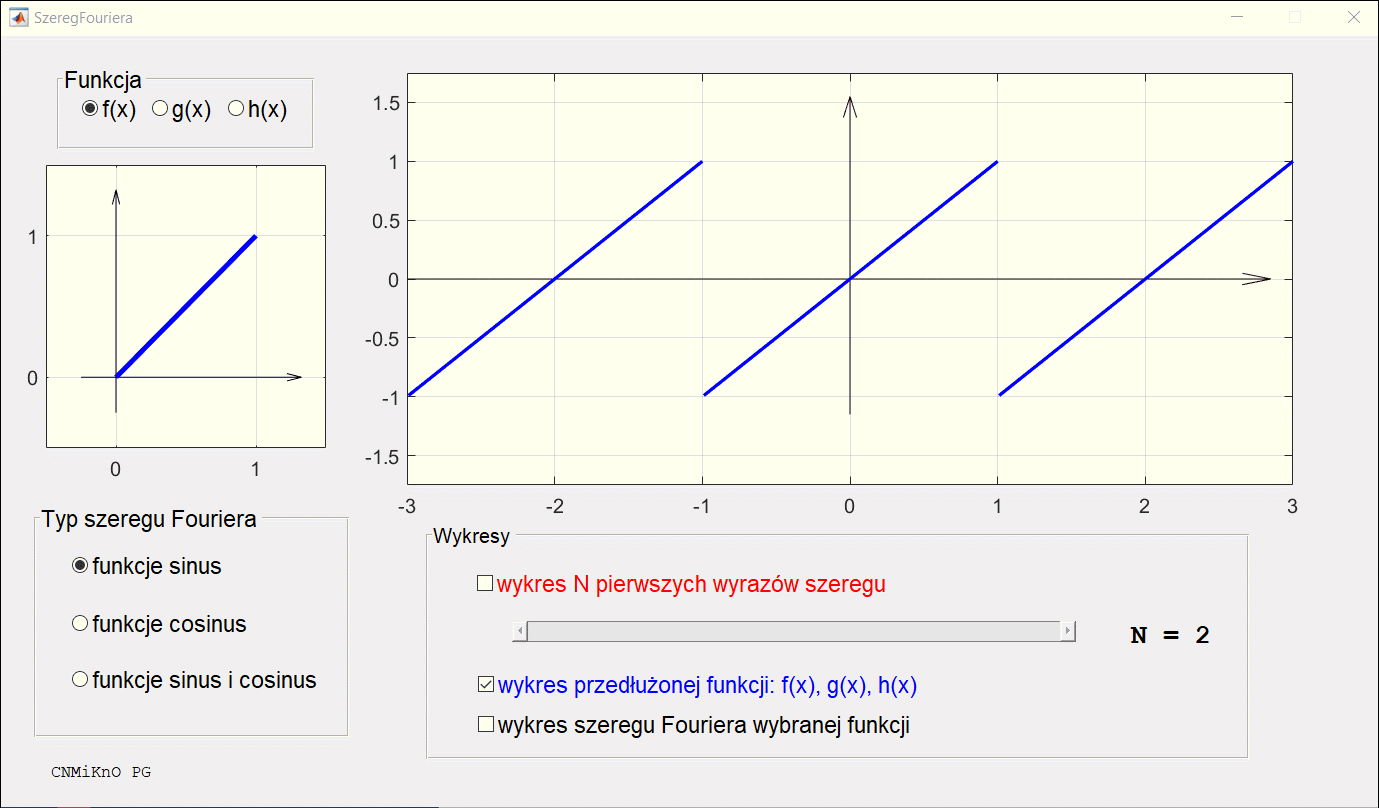##### Direction field and graphs of ODE solutions

App draws a direction field and solution curves of a first order ordinary differential equation.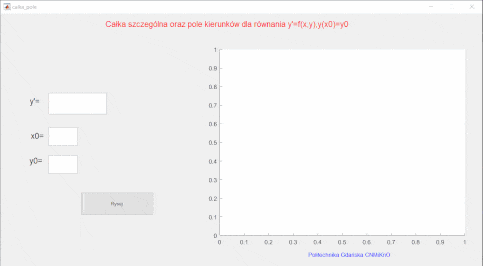##### Stability of a system of ODEs

App supports the analysis of stability of a system of ODEs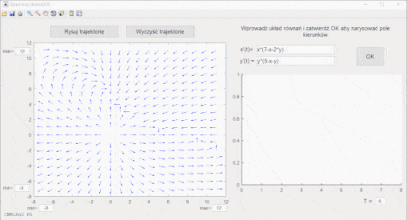##### Harmonic Oscillator

App showing the behavior of a harmonic oscillator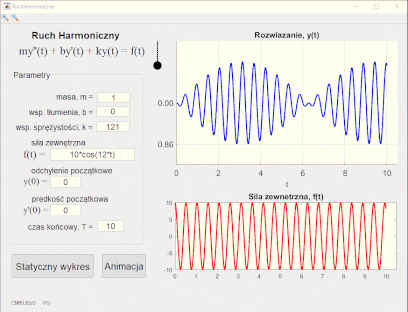Licence CC BY-SA
Mathematics Teaching and Distance Learning Center
Gdańsk University of Technology